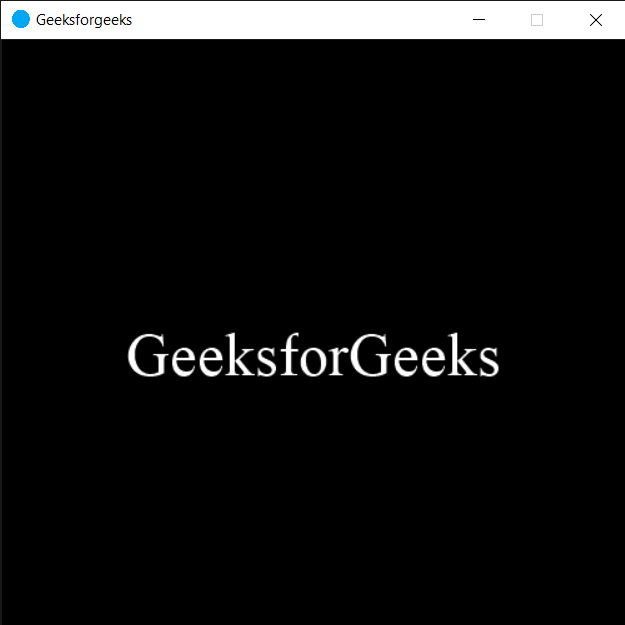# PYGLET – Setting Window Icon

In this article we will see how we can set the window icon in PYGLET module in python. Pyglet is easy to use but powerful library for developing visually rich GUI applications like games, multimedia etc. A window is a “heavyweight” object occupying operating system resources. Windows may appear as floating regions or can be set to fill an entire screen (fullscreen). An icon is a small graphical representation of a program or file. Icons help users quickly identify the type of file represented by the icon. Icon appear on the left hand side of the title of window.
We can create a window with the help of command given below

` pyglet.window.Window(width, height, title)`

In order to create window we use set_icon method with the window object
Syntax : window.set_icon(img)
Argument : It takes pyglet.image object as argument
Return : It returns None

Below is the implementation

## Python3

 `# importing pyglet module` `import` `pyglet` `import` `pyglet.window.key`   `# width of window` `width ``=` `500`   `# height of window` `height ``=` `500`   `# caption i.e title of the window` `title ``=` `"Geeksforgeeks"`   `# creating a window` `window ``=` `pyglet.window.Window(width, height, title)`   `# text ` `text ``=` `"GeeksforGeeks"`   `# creating a label with font = times roman` `# font size = 36` `# aligning it to the center` `label ``=` `pyglet.text.Label(text,` `                          ``font_name ``=``'Times New Roman'``,` `                          ``font_size ``=` `36``,` `                          ``x ``=` `window.width``/``/``2``, y ``=` `window.height``/``/``2``,` `                          ``anchor_x ``=``'center'``, anchor_y ``=``'center'``)`   `# on draw event` `@window``.event` `def` `on_draw():` `    `  `    ``# clearing the window` `    ``window.clear()` `    `  `    ``# drawing the label on the window` `    ``label.draw()` `    `  `# key press event    ` `@window``.event` `def` `on_key_press(symbol, modifier):` `    `  `    ``# key "C" get press` `    ``if` `symbol ``=``=` `pyglet.window.key.C:` `        `  `        ``# close the window` `        ``window.close()`   `# image for icon` `img ``=` `image ``=` `pyglet.resource.image("logo.png")`   `# setting image as icon` `window.set_icon(img)` `               `  `# start running the application` `pyglet.app.run()`

Output :